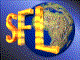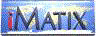| iMatix home page | << | < | > | >>SFLVersion 2.11

### strt2descr

```#include "sflstr.h"
DESCR *
strt2descr (
char **table)
```

#### Synopsis

Converts a table of strings into a single block of memory. The input table consists of an array of null-terminated strings, terminated in a null pointer. Returns the address of a DESCR block defined as: "typedef struct {size_t size; byte *data} DESCR;". Allocates the descriptor block using the mem_alloc() function; you must free it using mem_free() when you are finished with it. The strings are packed into the descriptor data field, each terminated by a null byte. The final string is terminated by two nulls. The total size of the descriptor is descr-> size + sizeof (DESCR). Note that if you omit the last null pointer in the input table, you will probably get an addressing error. Returns NULL if there was insufficient memory to allocate the descriptor block.

#### Source Code - (sflstr.c)

```{
DESCR
*descr;                         /*  Allocated descriptor             */
char
*descr_ptr;                     /*  Pointer into block               */
size_t
descr_size;                     /*  Size of table                    */
int
string_nbr;                     /*  Index into string table          */

ASSERT (table);

/*  Calculate the size of the descriptor                                 */
descr_size = 1;                     /*  Allow for final null byte        */
for (string_nbr = 0; table [string_nbr]; string_nbr++)
descr_size += strlen (table [string_nbr]) + 1;

/*  Allocate a descriptor and fill it with the strings                   */
descr = mem_alloc (descr_size + sizeof (DESCR));
if (descr)
{
descr-> size = descr_size;
descr-> data = (byte *) descr + sizeof (DESCR);
descr_ptr    = (char *) descr-> data;

for (string_nbr = 0; table [string_nbr]; string_nbr++)
{
size_t descr_len = strlen (table [string_nbr]) + 1;
strncpy (descr_ptr, table [string_nbr], descr_len);
descr_ptr += descr_len;
}
*descr_ptr = '\0';              /*  Add a null string                */
}
return (descr);
}
```

 | << | < | > | >>Copyright © 1996-2000 iMatix Corporation